# Bose-Einstein statistics

Bose statistics

Quantum statistics used in systems of identical particles with integral spin (in the unit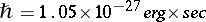). Presented by S. Bose and A. Einstein in 1924. According to these statistics an arbitrary number of particles may be found in each quantum state. It was shown by W. Pauli that the type of quantum statistics is directly related to the particle spin, since the population of particles with an integral spin obeys the Bose–Einstein statistics, while those with half-integral spin obey the Fermi–Dirac statistics.

The state of a system of many particles is defined in quantum mechanics by a wave function which, in the case of identical particles, may be symmetric with respect to the rearrangement of any pair of particles (for particles with integral spin) or anti-symmetric (for particles with half-integral spin). For a system of particles obeying the Bose–Einstein statistics the states are described by symmetric wave functions. This is a second, equivalent, formulation of the Bose–Einstein statistics. Systems of a large number of particles obeying Bose–Einstein statistics are known as Bose systems (e.g. a Bose gas).

For an ideal quantum gas, i.e. for a system of identical particles with masswhich do not interact, in a cube of volumethe quantum energy levels for single particles are given by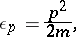whereare the eigen values of the momentum of the individual particle: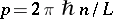, whereis a vector with integer (positive, negative or zero) components.

The quantum state of an ideal gas is defined by specifying the population of occupation numbers of the levels, where eachis the number of particles in the one-particle state. For Bose systems.

For large systems the energy levels are very densely located and tend to form a continuous spectrum as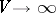. Let the levels be grouped by small cells, containing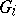levels per cell. To each cell corresponds the average energy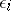, and the numberis assumed to be very large. The state of the system is defined by the selection, whereis the sum of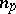taken over the levels of the cell. The statistical weight, that is, the number of different particle distributions over the cells, is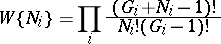(1)

and defines the probability of the particle distribution over the cells, characterized by the occupation numbers.

The most-probable distribution corresponding to a given energy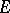and number of particles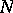:(2)

is found from the extremum of (1) under the complementary conditions (2). The corresponding average occupation numbers are(3)

whereis the chemical potential,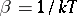,is the Boltzmann constant (a universal constant), andis the absolute temperature. The values ofandcan be found from the conditions (2).

The entropy of the system is defined as the logarithm of the statistical weight (1) for the most-probable distribution (3):(4)

Other thermodynamic functions may be derived from the entropy and the average energy.

The usual approach to Bose–Einstein statistics consists in employing the large canonical Gibbs distribution for the probabilityof the quantum levelof the entire system being filled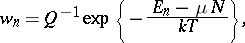(5)

where(6)

is the sum over states, or partition function, and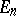are the energy levels of the entire system. Thus, for instance, for an ideal Bose gas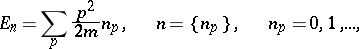and from equations (5) and (6) formula (3) for the occupation numbers, and formula (4) for the entropy can be found, without the use of combinatorial analysis. Such an approach is particularly important in the case of non-ideal Bose systems, when the use of Bose–Einstein statistics cannot be reduced to a simple combinatorial problem. In such a case the requirements of Bose–Einstein statistics can be met if for the Hamilton operator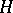the representation of second quantization is used, in which its action is defined on the space of symmetric wave functions or on the space of occupation numbers. The sum over states will then be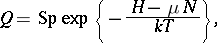whereis the operator for the number of particles; this sum may be used to find all the thermodynamic functions of the Bose system.

How to Cite This Entry:
Bose-Einstein statistics. Encyclopedia of Mathematics. URL: http://encyclopediaofmath.org/index.php?title=Bose-Einstein_statistics&oldid=22173
This article was adapted from an original article by D.N. Zubarev (originator), which appeared in Encyclopedia of Mathematics - ISBN 1402006098. See original article